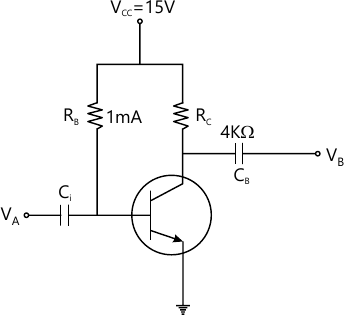MORE IN Analog and Digital Electronics
VTU Computer Science (Semester 3)
Analog and Digital Electronics
December 2013
Total marks: --
Total time: --
INSTRUCTIONS
(1) Assume appropriate data and state your reasons
(2) Marks are given to the right of every question
(3) Draw neat diagrams wherever necessary

1 (a) Explain the criteria for selecting a suitable operating point and factors affecting the stability.
8 M
1 (b) Determine the operating point for a fixed bias circuit shown in Fig.Q1(b). Given β=100 and VBE=07V. Draw the load line7 M
1 (c) Explain transistor as a switch.
5 M

2 (a) What are the differences between BJTs and FETs?
6 M
2 (b) Explain the working of a CMOS inverter.
4 M
2 (c) Explain the construction, working and principle of operation of an n-channel JFET.
10 M

3 (a) Explain the classification of optoelectronic devices.
6 M
3 (b) Define the following terms:
(i) Responsivity
(ii) NEP
(iii) Response time
(iv) Quantum efficiency
4 M
3 (c) Explain the working of cathode ray tube with a neat diagram. What are the advantages and disadvantages of CRT?
10 M

4 (a) What is the need for cascading amplifier? Explain two stage cascaded amplifier with a block diagram.
8 M
4 (b) Derive the expression for:
(i) Current gain
(ii) Input impedance
(iii) Voltage gain
12 M

5 (a) Briefly explain the classification amplifier based on input and output parameter of interest [Vi, Vo, Ii and io]
12 M
5 (b) With a neat diagram, explain amplifier with negative feed back.
4 M
5 (c) Calculate the values of harmonic distortion components for an output signal having an amplitude of 5V at fundamental frequency, second harmonic component of 0.05V and find total harmonic distortion.
4 M

6 (a) What are necessary conditions for loop gain and phase shift for sustained oscillations according to Barkhausen criterion?
4 M
6 (b) Explain the working of an Astable multivibrator using IC 555 timer with circuit diagram and relevant waveforms.
8 M
6 (c) Explain the working of RC low pass RC high pass circuit.
8 M

7 (a) Explain the steps involved in custom design of mains transformer.
6 M
7 (b) What are SMPS? Compare linear power supplies with SMPS.
7 M
7 (c) Explain the working of three terminal voltage regulators.
7 M

8 (a) What should be the slew rate choosen for an OPAMP as an inverting amplifier configuration with gain of 10 when input is a sinusoidal signal with peak to peak value of 2V and highest frequency expected is 50 Hz?
4 M
8 (b) Explain the working of an OPAMP window comparator with circuit diagram.
8 M
8 (c) Explain the lead and tag type of phase shifter.
8 M

More question papers from Analog and Digital Electronics